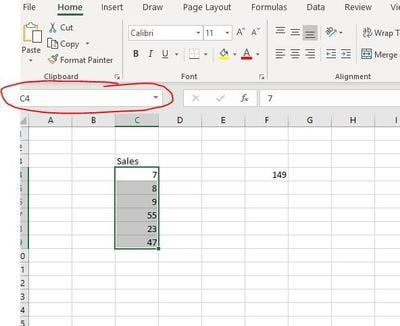Home >  Blog >  Introduction to naming ranges in Excel.

# Introduction to naming ranges in Excel.

Posted by Datasift on 26 March 2019

"Do what?", I hear you ask. Well, how many times have you had to jump around Excel trying to find out what an Excel formula is actually doing?

Lets look at a simple example. =SUM(\$C\$4:\$C\$9) this is adding up a range of cells. However, it does not tell you what the cells contain - you have to find the range and look at the context.

If we highlight the range by dragging down and then click in the name box (circled in red) we can name the range "Sales".So now our formula TELLS us what is going on because we can refer to the range to create the formula =SUM(Sales) - just imagine how much easier this is to understand what is going on.

Try it for constants like GSTRate, USDExchRate - it will really make a difference to other people's understanding of your work, too!

Author: Datasift
 Tags: Top Tip